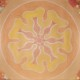Last Updated on 2022-05-18 by 明心

《幾何原本》（Στοιχεῖα，Stoicheia，Elements），文明暢銷書總榜單無敵暢銷書，連續300年長踞暢銷榜第一，連續2000年長踞暢銷榜第二，在今日卻罕為人知的曠世巨著。

• 哲學、數學、物理、科學、文明聯合強力推薦！！
• 《世界暢銷書 Top 10》，雄踞排行榜超過154451天！！！
• 笛卡兒、牛頓、歐拉、高斯、愛因斯坦等人衷心推薦。
• 入選文明影響力指南，被評為「撬動世界的槓桿」。

## 名字都很像的古希臘哲學家

• 亞歷山卓的歐幾里得（Euclid）：今天的主角，詳情後續。
• 阿基米德（Archimedes）：辨識真假皇冠、用棍子撬地球的裸奔愛好者。
• 亞里斯多德（Aristotle）：柏拉圖的弟子，亞歷山大大帝的老師，牛頓出道前，西方知識體系最具影響力的人物之一。
• 墨伽拉的歐幾里得（Euclid of Megara）：古希臘哲學家，以「自稱正撒謊者能否講真話」悖論著名。

### 教你如何高EQ應對無理的上司

EQ的歐幾里得偷偷地罵：「想偷懶，食屎啦你！」（No Way!）

## 《幾何原本》

### 只能從源頭出發

• 定義：一件事物基礎屬性的描述、說明。
• 公設：從經驗中，總結出的幾何學常識、事實。
• 公理：顯而易見、不證自明的斷言。

### 23個定義

1. A point is that which has no part.（點是沒有部分的）
2. A line is breadthless length.（線只有長度、沒有寬度）
3. The ends of a line are points.（一條線的兩端是點）
4. A straight line is a line which lies evenly with the points on itself.（一條直線是沿一定方向，及其反方向的點所鋪成）
5. A surface is that which has length and breadth only.（面只有長度和寬度）
6. The edges of a surface are lines.（一個面的邊緣是線）
7. A plane surface is a surface which lies evenly with the straight lines on itself.（平面是直線自身的均勻分布）
8. A plane angle is the inclination to one another of two lines in a plane which meet one another and do not lie in a straight line.（平面角，是在一平面內兩條直線相交，所形成的傾斜程度）
9. And when the lines containing the angle are straight, the angle is called rectilinear.（當平面角的角度是筆直時，則為平角180°）
10. When a straight line standing on a straight line makes the adjacent angles equal to one another, each of the equal angles is right, and the straight line standing on the other is called a perpendicular to that on which it stands.（當一直線站立於另一條直線，使得形成的角度，相等於形成的另一角度，則兩個角度都可以稱為直角90°，而其中一條線可稱為另一條直線的垂線）
11. An obtuse angle is an angle greater than a right angle.（鈍角是大於直角的角）
12. An acute angle is an angle less than a right angle.（銳角是小於直角的角）
13. A boundary is that which is an extremity of anything.（邊界是事物之邊緣）
14. A figure is that which is contained by any boundary or boundaries.（圖是由一個或多個邊界所構成）
15. A circle is a plane figure contained by one line such that all the straight lines falling upon it from one point among those lying within the figure equal one another.（圓是由一條線包圍著的平面圖形，內部有一點，與這條線上任何一點，所連成的線段都相等）
16. And the point is called the center of the circle.（此點為圓心）
17. A diameter of the circle is any straight line drawn through the center and terminated in both directions by the circumference of the circle, and such a straight line also bisects the circle.（直徑是通過圓心，且端點在圓上的任意線段，該線段將圓分成兩等分）
18. A semicircle is the figure contained by the diameter and the circumference cut off by it. And the center of the semicircle is the same as that of the circle.（半圓是直徑，和被它所切割的圓弧，所形成的圖形。半圓之圓心和原本之圓心相同）
19. Rectilinear figures are those which are contained by straight lines, trilateral figures being those contained by three, quadrilateral those contained by four, and multilateral those contained by more than four straight lines.（直線圖形，是由線段首尾-首尾相連，所形成的圖形。三角形由三條線段所圍成，四邊形由四條線段所圍成，多邊形由四條以上的線段所圍成）
20. Of trilateral figures, an equilateral triangle is that which has its three sides equal, an isosceles triangle that which has two of its sides alone equal, and a scalene triangle that which has its three sides unequal.（三角形中，三條邊相等之三角形為正三角形，兩條邊相等的為等腰三角形，各邊都不相等的為不等邊三角形）
21. Further, of trilateral figures, a right-angled triangle is that which has a right angle, an obtuse-angled triangle that which has an obtuse angle, and an acute-angled triangle that which has its three angles acute.（此外，三角形中有一個直角的為直角三角形，有一個角是鈍角的為鈍角三角形，三個角都是銳角的為銳角三角形）
22. Of quadrilateral figures, a square is that which is both equilateral and right-angled; an oblong that which is right-angled but not equilateral; a rhombus that which is equilateral but not right-angled; and a rhomboid that which has its opposite sides and angles equal to one another but is neither equilateral nor right-angled. And let quadrilaterals other than these be called trapezia.（在四邊形中，四個邊相等，且四個角都是直角的為正方形；四個角是直角，但四個邊不完全相等的是矩形；四個邊相等，角不是直角的為菱形；兩組對邊、兩組對角分別相等的，是平行四邊形；一組對邊平行、一組對邊不平行的，為梯形）
23. Parallel straight lines are straight lines which, being in the same plane and being produced indefinitely in both directions, do not meet one another in either direction.（平行直線，是在同一平面，向兩個方向無限延長，卻無法相交的直線）

### 5個公設

• To draw a straight line from any point to any point.（從一點向另一點可引一條直線）
• To produce a finite straight line continuously in a straight line.（任意線段能無限延伸成一條直線）
• To describe a circle with any center and radius.（給定任意線段，可以以其一個端點作為圓心，該線段作為半徑作一個圓）
• That all right angles equal one another.（所有直角都相等）
• That, if a straight line falling on two straight lines makes the interior angles on the same side less than two right angles, the two straight lines, if produced indefinitely, meet on that side on which are the angles less than the two right angles. （若兩條直線都與第三條直線相交，並且在同一邊的內角之和小於兩個直角，則這兩條直線在這一邊必定相交）

### 5個公理

• Things which equal the same thing also equal one another.（與同一事物相等的事物相等，即若A=B且B=C，則A=C）
• If equals are added to equals, then the wholes are equal.（相等的事物加上相等的事物仍然相等，即若A=B，則A+C=B+C）
• If equals are subtracted from equals, then the remainders are equal.（相等的事物減去相等的事物仍相等，即若A=B，則A-C=B-C）
• Things which coincide with one another equal one another.（一個事物與另一事物重合，則它們相等）
• The whole is greater than the part. （整體大於局部）

### 命題I.1

1. 以A為圓心，以AB為半徑畫圓BCD（根據：公設3）
2. 以B為圓心，以BA為半徑畫圓ACE（根據：公設3）
3. 從兩圓的交點C，連結點A、C，畫出CA、CB。（根據：公設1）
4. 由於點A是圓BCD的圓心，所以AC=AB。（根據：定義15）
5. 由於點B是圓ACE的圓心，所以BC=AB。（根據：定義15）
6. 由於AC=AB且BC=AB，所以AC=BC。（根據：公理1）

### 命題I.2

1. 連點A和點B，成為線段AB。（根據：公設1）
2. 以有限線段AB為一邊，做一個正三角形ABD（根據：命題1）
3. 延長DA成為直線DE，延長直線DB成為直線DF。（根據：公設2）
4. 以B為圓心，BC為半徑畫圓CGH。（根據：公設3）
5. 以D為圓心，DG為半徑畫圓GKL。（根據：公設3）
6. 由於點B是圓CGH的圓心，所以BC=BG。（根據：定義15）
7. 由於點D是圓GKL的圓心，所以DL=DG。（根據：定義15）
8. 同時因為DA=DB，所以AL=BG。（根據：公理3）
9. 由6可知，BC=BG，所以AL、BC皆等於BG。（根據：公理1）

### 幾何原本最重要的精神

1.Jenny

天呀！這本書很有意思～我超認真看的！
我非常喜歡數學，從小只有數學比較好
長大後發現 邏輯在工作生活中也是好重要～（比方說需要直覺的機關等等

這邊講的很好
我們今日的教育鮮少提及《幾何原本》背後的精神
反倒著重在其中的命題、解法，成為國小、國中的教學內容，這是十分可惜的事情。

若是學生能深刻的經驗數學之美，這樣的教育才是具有生命力的教育。

真的很希望不要再有人說 學數學幹嘛 我又沒有要當數學家XDDD

•明心

感受美，在我們教育和社會認同中，
真的是比較欠缺的一塊。

噢，現在的”性向測驗”中，居然還有讓人選
“感覺最和諧的顏色”這種題目
來評量一個人的美感程度……
反倒是捨本逐末了

2.Gordon

某種謹慎、緊密、環環相扣的美
直到微積分與無線開始加入XD